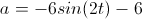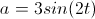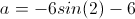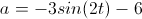# Calculus 1 : How to find acceleration

## Example Questions

← Previous 1 3 4 5 6 7 8 9 34 35

### Example Question #1 : How To Find Acceleration

The position vector of an object moving in a plane is given by r(t)=3t4i + 6t2j. Find its acceleration when t=1.

36i + 12j
3i + 2j
36i - 12j
6i + 2j
3i + 6j
Explanation:

To find acceleration at time t, we have to differentiate the position vector twice.

Differentiating the first time gives the velocity:

v(t) = r'(t) = 12t3i + 12tj

Differentiating a second time gives the accelaration:

a(t) = r''(t) = 36t2i + 12j

Plug in t=1 to solve for the final answer:

a(1) = r''(1) = 36i + 12j

### Example Question #1 : How To Find Acceleration

The relative displacement (in meters) of a particle at time t is defined by the function f(t) = 4t2 * ln(t)

What is the instantaneous acceleration of the particle at t = 3.5?

49.08 m2

61.39 m2

22.02 m2

55.37 m2

22.02 m2

Explanation:

The instantaneous acceleration is found by taking the 2nd derivative of the function and applying thereto the desired variable parameter. First let us calculate the 1st derivative:

f(t) = 4t2 * ln(t) will require us to apply the product rule; therefore:

f'(t) = 8t * ln(t) + 4t2 * (1/t), which reduces to: 8t*ln(t) + 4t

Taking the second derivative (applying the product rule to the first value), we get:

f''(t) = 8 * ln(t) + 8t * (1/t) + 4, which simplifies to: 8 * ln(t) + 8 + 4 = 8 * ln(t) + 12

The instantaneous acceleration at t = 3.5 is found by solving:

f''(3.5) = 8 * ln(3.5) + 12 = 22.02210374796294 (approx.) or 22.02

### Example Question #3 : How To Find Acceleration

What is the instantaneous acceleration at time t = 4 of a particle whose positional equation is represented by s(t) = 5t3 – 24t2 + 44?

72

56

60

70

48

72

Explanation:

The instantaneous acceleration is represented by the second derivative of the positional equation. Let's first calculate the velocity then the acceleration:

v(t) = s'(t) = 15t2 – 48t

a(t) = v'(t) = s''(t) = 30t – 48

a(4) = 30 * 4 – 48 = 72

### Example Question #1 : How To Find Acceleration

What is the instantaneous acceleration at time t = π of a particle whose positional equation is represented by s(t) = 5sin(4t) + cos(2t)?

–84

–80

–76

–4

–4

Explanation:

The instantaneous acceleration is represented by the second derivative of the positional equation. Let's first calculate the velocity then the acceleration:

s(t) = 5sin(4t)+ cos(2t)?

v(t) = s'(t) = 20cos(4t) – 2sin(2t)

a(t) = v'(t) = s''(t) = –80sin(4t) – 4cos(2t)

a(π) = –80sin(4π) – 4cos(2π) = –80sin(0) - 4cos(0) = 0 – 4 = –4

### Example Question #2 : How To Find Acceleration

What is the instantaneous acceleration at time t = π/2 of a particle whose positional equation is represented by s(t) = 5sin(t/2 + π)?

5√2/4

5√2/8

5√2/2

–5√2/4

5√2/8

Explanation:

The instantaneous acceleration is represented by the second derivative of the positional equation.  Let's first calculate the velocity then the acceleration:

s(t) = 5sin(t/2 + π)?

v(t) = s'(t) = (5/2)cos(t/2 + π)

a(t) = v'(t) = s''(t) = –(5/4)sin(t/2 + π)

We know that sin(x) = –sin(x + π); therefore, sin(t/2 + π) = –sin(t/2). Let's rewrite a(t) as (5/4)sin(t/2).

The acceleration is therefore a(π/2) = (5/4)sin(π/2/2) = (5/4)sin(π/4) = 5/4 * (1/√2) = 4/(4√2) = 5√2/8

### Example Question #6 : How To Find Acceleration

Find the accelerationof a particle with a position given by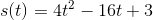at time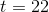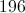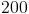Explanation:

Acceleration is given by the second derivative of position.  So for this equation, the second derivative is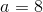.  The acceleration is constant through time, so the answer is simply 8.

### Example Question #1 : How To Find Acceleration

Jerk is defined as the rate of change of acceleration.  Find the function of jerk with respect to time for a particle with position given by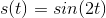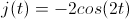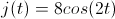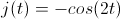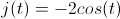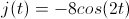Explanation:

Since jerk is the rate of change of acceleration, and acceleration is the second derivative of position, the answer is to find the third derivative of position.  Use the chain rule each time.

### Example Question #8 : How To Find Acceleration

The position of a particle is given by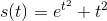.  Find the acceleration of the particle when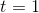.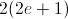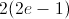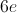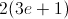Explanation:

The acceleration of a particle is given by the second derivative of the position function.  We are given the position function as.

The first derivative (the velocity) is given as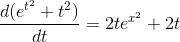.

The second derivative (the acceleration) is the derivative of the velocity function.  This is given as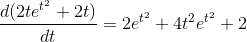.

Evaluating this atgives us the answer.  Doing this we get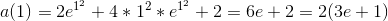.

### Example Question #9 : How To Find Acceleration

Suppose a particle travels in a circular motion in the xy-plane with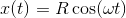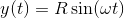for some constants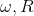. Notice that this is circular because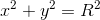.

What is the total magnitude of the acceleration,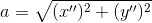?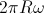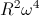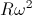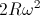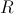Explanation:

We know that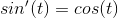and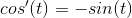so the acceleration components are: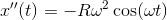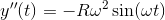Plugging these into the formula for the acceleration and again recognizing that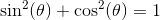, we get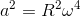, or, after the square root,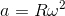.

### Example Question #10 : How To Find Acceleration

Find the acceleration of a particle at any given time if its velocity is given by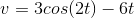?